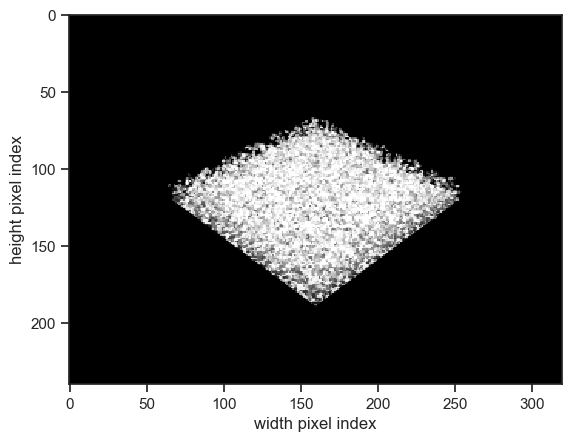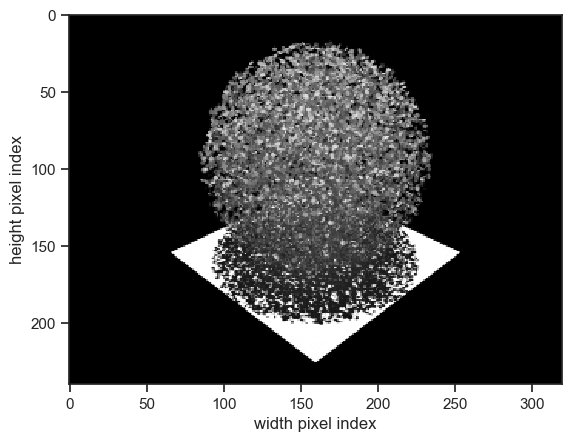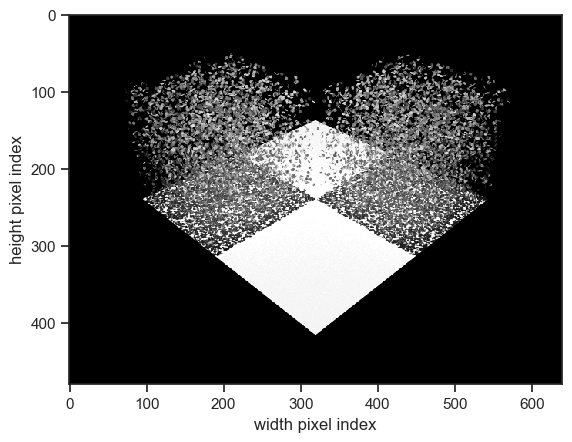Go to notebook file

:

%reload_ext eradiate.notebook.tutorials


Last updated: 2023-06-22 19:43 (eradiate v0.23.2rc2.post1.dev5+gedede817.d20230622)

# Building abstract canopies#

Overview

This tutorial provides an overview of the different ways of specifying abstract canopies, based on floating disks, arranged into different geometric shapes, such as cube, cone and ellipsoid (sphere). Canopies based on triangulated meshes are not covered in this tutorial.

Prerequisites

What you will learn

• How to specify canopies and their constituents.

## Imports#

As for all tutorials the first step is to import all necessary classes and create aliases for convenience:

:

%load_ext eradiate

import numpy as np
import matplotlib.pyplot as plt

from eradiate import unit_registry as ureg


Since we will be performing simulations on scenes without an atmosphere, we select the monochromatic (line-by-line) mode. Next, we set up convenience functions for plotting BRF results and visualising camera renders.

:

def show_camera(
exp,
measure_id,
robust=True,
vmin=None,
vmax=None,
):
"""
Display the output of a monochromatic camera measure.
"""
_, ax = plt.subplots(1,1)
ax=ax,
origin="upper",
cmap="Greys_r",
vmin=vmin,
vmax=vmax,
robust=robust,
)
ax.set_aspect(1)
plt.show()
plt.close()

def show_brf(exp, measure_id):
"""
Display the BRF output of a distant radiance measure.
"""
_, ax = plt.subplots(1,1)
with plt.style.context({"lines.linestyle": ":", "lines.marker": "."}):
exp.results[measure_id]["brf"].squeeze(drop=True).plot(ax=ax, x="vza")
plt.show()
plt.close()


## Abstract leaf cloud#

We start with the scene created in the 3D simulation basics tutorial: a Lambertian surface topped with a an abstract leaf cloud consisting of disks.

:

lambertian_surface=ertsc.surface.BasicSurface(
shape=ertsc.shapes.RectangleShape(edges=10.0 * ureg.m),
bsdf=ertsc.bsdfs.LambertianBSDF(reflectance=0.5),
)

homogeneous_canopy = ertsc.biosphere.DiscreteCanopy.homogeneous(
l_vertical=1.0 * ureg.m,
l_horizontal=10.0 * ureg.m,
lai=2.0,
)


Next, we create a perspective camera to visualise the scene:

:

camera_oblique = ertsc.measure.PerspectiveCameraMeasure(
id="camera_oblique",
origin=[15, 15, 15] * ureg.m,
target=[0, 0, 0] * ureg.m,
up=[0, 0, 1],
film_resolution=(320, 240),
spp=512,
)


Now we create an experiment object. We use the CanopyExperiment, designed to simulate radiative transfer in scenes with 3D canopies without an atmosphere. We leave the illumination unset: it defaults to a directional emitter with at the zenith.

:

exp = eradiate.experiments.CanopyExperiment(
surface=ertsc.bsdfs.LambertianBSDF(reflectance=0.5),
canopy=homogeneous_canopy,
measures=camera_oblique,
)


We run the simulation and use the convenience function defined above to visualise the result.

:

eradiate.run(exp)
show_camera(exp, "camera_oblique")## More options#

Eradiate supports more complex geometries for abstract (leaf cloud-based) canopies. This requires a manual initialisation of the DiscreteCanopy object.

A DiscreteCanopy instance holds a list of InstancedCanopyElement objects. Each InstancedCanopyElement object contains an explicit canopy element such (e.g. a LeafCloud object) and holds list of positions, at which instances (i.e. clones) of the canopy element will be created. Cloning several times the same object with this mechanism is cheap.

Here,we define a single instance position that positions a spherical leaf cloud above of the surface:

:

spherical_leaf_cloud = ertsc.biosphere.LeafCloud.sphere(
n_leaves = 10000,
leaf_reflectance=0.5,
leaf_transmittance=0.5,
)

canopy_sphere = ertsc.biosphere.DiscreteCanopy(
instanced_canopy_elements=ertsc.biosphere.InstancedCanopyElement(
id="sphere",
canopy_element=spherical_leaf_cloud,
instance_positions=[(0, 0, 5.0)] * ureg.m
),
size=[10.0, 10.0, 10.0] * ureg.m,
)


Since this canopy has a larger vertical extent, we update our camera parameters so that the canopy fits well within its field of view:

:

camera_oblique = ertsc.measure.PerspectiveCameraMeasure(
id="camera_oblique",
origin=[15, 15, 15] * ureg.m,
target=[0, 0, 3.0] * ureg.m,
up=[0, 0, 1],
film_resolution=(320, 240),
spp=512,
)


Again, we create an experiment, run it and visualise the results.

:

exp = eradiate.experiments.CanopyExperiment(
surface=ertsc.bsdfs.LambertianBSDF(reflectance=0.8),
canopy=canopy_sphere,
measures=camera_oblique,
)
show_camera(exp, "camera_oblique")More kinds of leaf cloud generators are available (see the list in the LeafCloud API reference).

## Abstract leaf cloud from definition files#

Warning

This section will create files on your machine, be sure to change file paths according to your local data structure or move files that might be overwritten!

It may be convenient to load leaf positions from a data file, e.g. if the canopy specification was created by a third party. For this reason, Eradiate’s abstract canopies can be specified from ASCII files. This is done using the DiscreteCanopy.leaf_cloud_from_files() constructor.

The input of this method consists of two files:

1. The first file specifies the parameters of the leaves (size, orientiation, position) inside the leaf cloud.

2. The second file specifies the locations at which leaf cloud instances are positioned (instance positions).

The ASCII definition file for the leaf cloud has to be formatted as follows:

• one leaf per line;

• each line holds seven floating point values:

• x, y and z coordinates of the leaf centre position (in metres);

• x, y and z coordinates of the leaf normal vector.

Let’s create a file with 5000 leaves at the root of the current working directory.

:

fname_cloud = "./tutorial_canopy_definition.txt"

with open(fname_cloud, "w") as outfile:
for i in range(5000):
rands = np.random.random(6)
px = (rands - 0.5) * 2 * 2
py = (rands - 0.5) * 2 * 2
pz = (rands - 0.5) * 2 * 2

nx = (rands - 0.5) * 2
ny = (rands - 0.5) * 2
nz = (rands - 0.5) * 2

outfile.write(f"0.05 {px} {py} {pz} {nx} {ny} {nz}\n")


The ASCII file for the instance positions simply holds three floating point values per line, which specify the x, y and z coordinates for the instances. Two copies of the leaf cloud positioned diagonally with respect to each other.

:

fname_instances = "./tutorial_canopy_instances.txt"

with open(fname_instances, "w") as outfile:
outfile.write("-2.0 2.0 2.0\n")
outfile.write("2.0 -2.0 2.0\n")


Next, we create a canopy object that uses these definition files:

:

canopy_files = ertsc.biosphere.DiscreteCanopy.leaf_cloud_from_files(
id="leaf_cloud",
leaf_cloud_dicts = [
{
"instance_filename": fname_instances,
"leaf_cloud_filename": fname_cloud,
"leaf_reflectance": 0.5,
"leaf_transmittance": 0.5,
"sub_id": "cube"
}
],
size=(8, 8, 4)*ureg.m
)


We update the camera and run an experiment to display our canopy:

:

camera_oblique = ertsc.measure.PerspectiveCameraMeasure(
id="camera_oblique",
origin=[10, 10, 10] * ureg.m,
target=[0, 0, 0.0] * ureg.m,
up=[0, 0, 1],
film_resolution=(640, 480),
spp=128,
)

surface=ertsc.bsdfs.LambertianBSDF(reflectance=0.8),
canopy=canopy_files,
measures=camera_oblique,
)This tutorial covered the creation of abstract canopies, but Eradiate also supports the specification of canopies using OBJ mesh files. This allows for a more realistic representation of real-world canopies. This will be covered in a dedicated tutorial; until then, interested users are referred to the documentation of the MeshTree class.